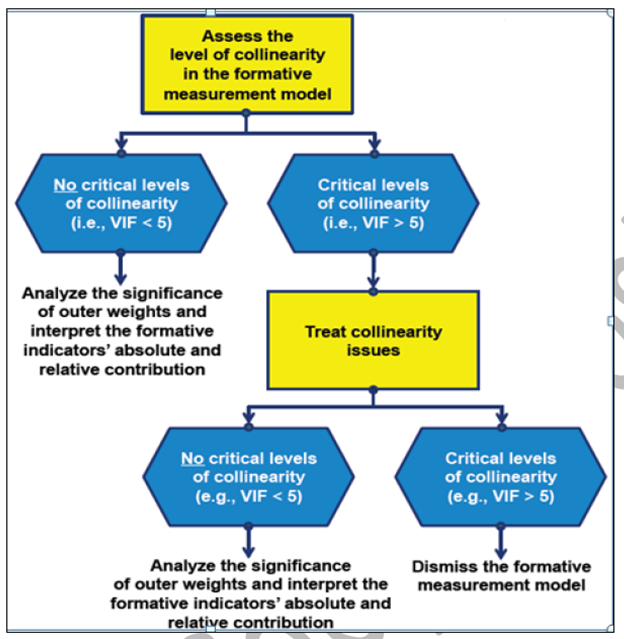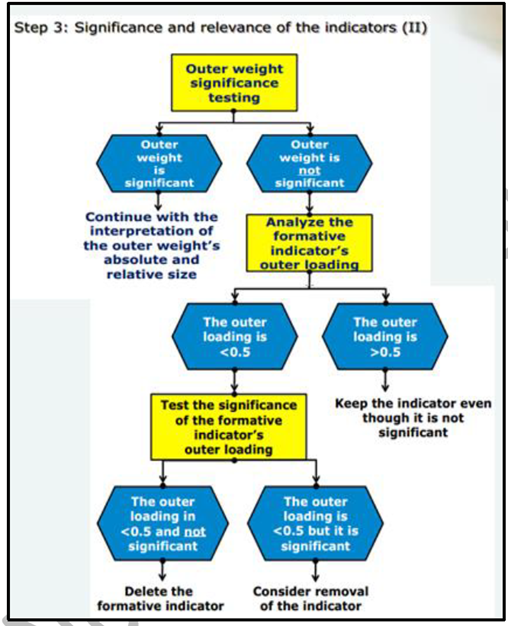# How to Validate Formative Model in SMART-PLS

## How to Validate Formative Model in SMART-PLS

Before Discussing How to Validate Formative Model, To Review What is Formative Construct and How does it differ from Reflective Construct, Click Here

Assessment of formative measurement model for collinearity issues

Since the indicators are not essentially inter-changeable, high correlations are not expected between indicators in formative measurement models. In fact, high correlations between two formative indicators are known as collinearity (Hair et al. 2014).

High levels of collinearity between formative indicators are problematic because they have an impact on the estimation of weights and their statistical significance.

In order to assess the level of collinearity in PLS-SEM, Variance Inflation Factor (VIF) is looked at. There are two widely accepted rules of thumbs:

• If VIF 5 or higher, it indicates a potential issue with collinearity problem (Hair, Ringle & Sarstedt, 2011).
• If VIF 3.3 or higher, it indicates a potential issue with collinearity problem (Diamantopoulos & Siguaw, 2006).Source: Primer on Partial Least Squares Structural Equation Modeling (PLS-SEM) Book (Hair et al., 2017)

## How to Validate Formative Model in SMART-PLS

The researchers must assess the significance and relevance of the formative indicators. For a formative (i.e. composite) construct, outer weight is an important criterion for evaluating the contribution of a formative indicator.

Hair et al. (2017) mention that outer weight is the result of a multiple regression with the latent variable scores as the dependent variable and the formative indicators as the independent variables.

The values of the outer weights can be obtained using bootstrapping technique (t-values are assessed for each indicator weight pointing towards the formative construct) and can therefore be used to determine each indicator’s relative contribution to the construct, or its relative importance to forming the construct.

When an indicator’s outer weight is non-significant but its outer loading is high (i.e. above 0.50), the indicator should be interpreted as absolutely important but not as relatively important. In this situation, the indicator would generally be retained.

However, when an indicator has a non-significant weight and the outer loading is below 0.50, the researchers should decide whether to retain or delete the indicator by examining its theoretical relevance and potential content overlap with other indicators of the same construct.Reference

Ramayah, T., Cheah, J., Chuah, F., Ting, H., & Memon, M. A. (2018). Partial least squares structural equation modeling (PLS-SEM) using smartPLS 3.0. In An Updated Guide and Practical Guide to Statistical Analysis. Pearson.